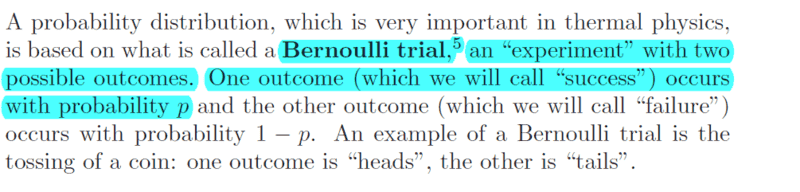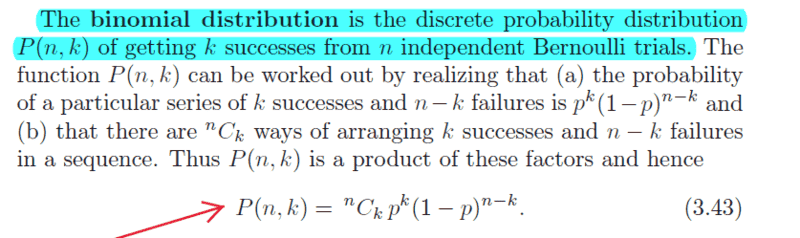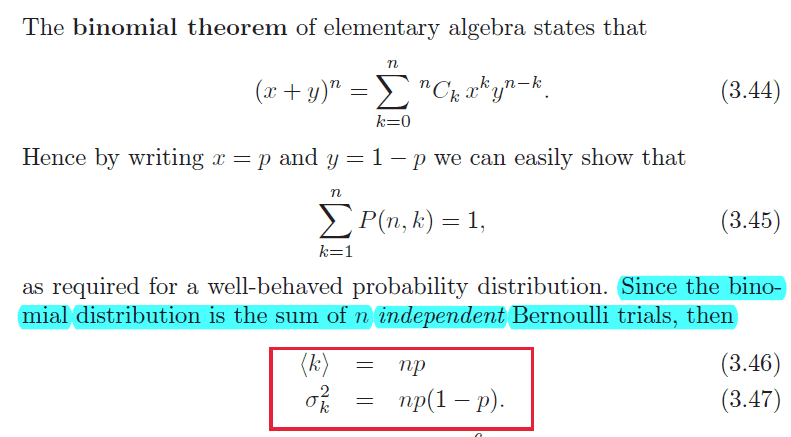# BInomial distribution

## Homework StatementI am not getting the above.
Let me calculate the probability of getting 2 successes from 5 Bernoulli trials.
There are total 10 possible outcomes as each trial has two possible outcomes.
The probability of getting one success is P(S1) = No. of successes / no. of total possible outcomes
= 5/10 = 1/2
The probability of getting another success = No. of successes / no. of total possible outcomes
= 4/9
So, the probability of getting two successes = 1/2(4/9)= 2/9
This doesn't match the answer given by the binomial distribution formula.
So, what is wrong here?I couldn't understand how is binomial distribution sum of n independent Bernoulli trials?
If I denote ith trial by ##X_i ##, then how can k i.e. no. of successes be sum of the trials i.e.∑##_i X_i##?

## The Attempt at a Solution

Last edited:

jambaugh
Gold Member
When you say there are 10 possible outcomes in 5 Bernoulli trials you are making a conceptual error. Each trial yields 2 outcomes so there are a total of ##2\cdot 2\cdot 2 ....\cdot 2 = 2^{10}## outcomes when you consider specific sequences. But there are 5+1 = 6 possible outcomes {0,1,2,3,4,5} when you consider the specific values of the binomial random variable K = number of successes might take on.

You're trying to derive the probability of getting 1 success by counting outcomes. Doing that you need to count the ##2^{10}## outcomes if these are each equally probable. Of those you need to count how many yield 1 success. That will be 5 choose 1 = 5 ways BUT this assumes each outcome is equally probable. That would be true if x=1/2 but Bernoulli trials needn't be 50-50. If you're talking coin flips then great. If you're talking winning the lottery vs losing then, this won't work.

You have to use independence of the trials. The derivation is pretty straight forward. In your example of the probability of 1 success you calculate the probability of exactly 1 success in a given sequence. For example in 5 trials getting [success, fail ,fail, fail, fail]. The probability of this will be ##p\cdot q\cdot q\cdot q\cdot q## where ##q=1-p## is the failure probability. You then multiply this by the count of how many rearrangements of the specific sequence there are.

In general you'll find the probability of getting ##k## successes (and ##n-k## failures) in a specific sequence will be ## p^k\cdot (1-p)^{n-k}## and there will be ## n## choose ##k## ways to rearrange that sequence (by choosing which ##k## of the ##n## trials will be the successes).

So the general binomial probability formula is: ##P(n,k) = \frac{n!}{k!(n-k)!} p^k(1-p)^{n-k}##
If you didn't follow my exposition, there's 100's online. Start at Wolfram or Wikipedia, or your textbook.